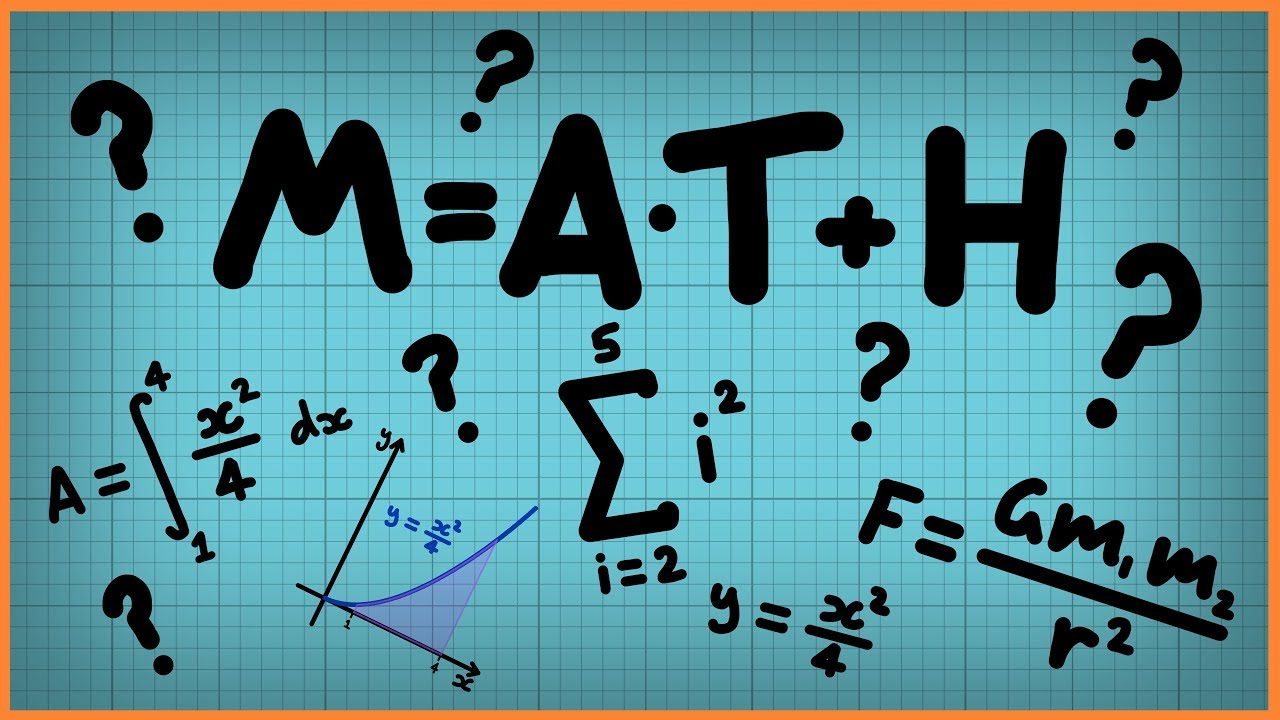Qalaxia Master Bot
0

I found an answer from byjus.com

Formula To Calculate Arc Length With Solved Examples

Example Questions Using the Formula for Arc Length. Question 1: Calculate the length of an arc if the radius of an arc is 8 cm and the central angle is 40°. Solution ...

For more information, see Formula To Calculate Arc Length With Solved ExamplesGuru Nanak
0

Given that

A and B are two opposite points on Earth

Subtended central angle \theta = 1 \degree 54'

Diameter of the earth S = 1.276 * 10^{7} m

Distance between the earth and moon = d

Step 1: Create a figure based on the details provided.Step 2: Calculating the distance between the earth and moon

Convert degrees minutes seconds to radians

\theta = 1 \degree 54' = 114' = 114* 60'' = 114 * 60 * 4.085* 10^{-6}

From the figure

AB is a diameter of the Earth

Assumption: AB is considering as a arc.

Arc length S = \theta d

d = \frac{S}{\theta}

d = \frac{1.276 * 10^{7}}{3.32* 10^{-2}}

d = 3.84 * 10^8 m

Hence, distance between the earth and moon d = 3.84 * 10^8 m